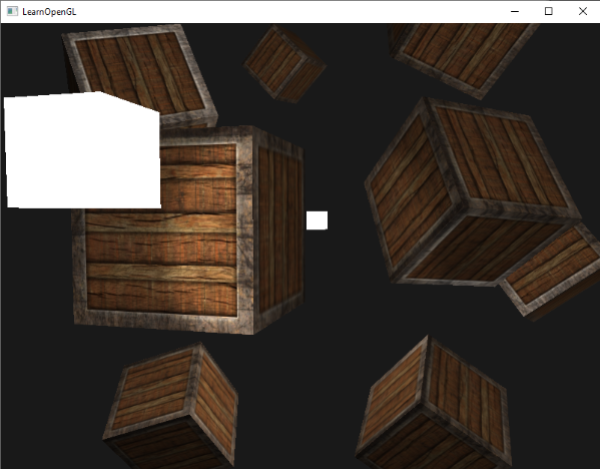# 多光源

GLSL中的函数和C函数很相似，它有一个函数名、一个返回值类型，如果函数不是在main函数之前声明的，我们还必须在代码文件顶部声明一个原型。我们对每个光照类型都创建一个不同的函数：定向光、点光源和聚光。

out vec4 FragColor;

void main()
{
// 定义一个输出颜色值
vec3 output;
// 将定向光的贡献加到输出中
output += someFunctionToCalculateDirectionalLight();
// 对所有的点光源也做相同的事情
for(int i = 0; i < nr_of_point_lights; i++)
output += someFunctionToCalculatePointLight();
// 也加上其它的光源（比如聚光）
output += someFunctionToCalculateSpotLight();

FragColor = vec4(output, 1.0);
}


## 定向光

struct DirLight {
vec3 direction;

vec3 ambient;
vec3 diffuse;
vec3 specular;
};
uniform DirLight dirLight;


vec3 CalcDirLight(DirLight light, vec3 normal, vec3 viewDir);


Important

vec3 CalcDirLight(DirLight light, vec3 normal, vec3 viewDir)
{
vec3 lightDir = normalize(-light.direction);
// 漫反射着色
float diff = max(dot(normal, lightDir), 0.0);
// 镜面光着色
vec3 reflectDir = reflect(-lightDir, normal);
float spec = pow(max(dot(viewDir, reflectDir), 0.0), material.shininess);
// 合并结果
vec3 ambient  = light.ambient  * vec3(texture(material.diffuse, TexCoords));
vec3 diffuse  = light.diffuse  * diff * vec3(texture(material.diffuse, TexCoords));
vec3 specular = light.specular * spec * vec3(texture(material.specular, TexCoords));
return (ambient + diffuse + specular);
}


## 点光源

struct PointLight {
vec3 position;

float constant;
float linear;

vec3 ambient;
vec3 diffuse;
vec3 specular;
};
#define NR_POINT_LIGHTS 4
uniform PointLight pointLights[NR_POINT_LIGHTS];


Important

vec3 CalcPointLight(PointLight light, vec3 normal, vec3 fragPos, vec3 viewDir);


vec3 CalcPointLight(PointLight light, vec3 normal, vec3 fragPos, vec3 viewDir)
{
vec3 lightDir = normalize(light.position - fragPos);
// 漫反射着色
float diff = max(dot(normal, lightDir), 0.0);
// 镜面光着色
vec3 reflectDir = reflect(-lightDir, normal);
float spec = pow(max(dot(viewDir, reflectDir), 0.0), material.shininess);
// 衰减
float distance    = length(light.position - fragPos);
float attenuation = 1.0 / (light.constant + light.linear * distance +
// 合并结果
vec3 ambient  = light.ambient  * vec3(texture(material.diffuse, TexCoords));
vec3 diffuse  = light.diffuse  * diff * vec3(texture(material.diffuse, TexCoords));
vec3 specular = light.specular * spec * vec3(texture(material.specular, TexCoords));
ambient  *= attenuation;
diffuse  *= attenuation;
specular *= attenuation;
return (ambient + diffuse + specular);
}


## 合并结果

void main()
{
// 属性
vec3 norm = normalize(Normal);
vec3 viewDir = normalize(viewPos - FragPos);

// 第一阶段：定向光照
vec3 result = CalcDirLight(dirLight, norm, viewDir);
// 第二阶段：点光源
for(int i = 0; i < NR_POINT_LIGHTS; i++)
result += CalcPointLight(pointLights[i], norm, FragPos, viewDir);
// 第三阶段：聚光
//result += CalcSpotLight(spotLight, norm, FragPos, viewDir);

FragColor = vec4(result, 1.0);
}


lightingShader.setFloat("pointLights.constant", 1.0f);


glm::vec3 pointLightPositions[] = {
glm::vec3( 0.7f,  0.2f,  2.0f),
glm::vec3( 2.3f, -3.3f, -4.0f),
glm::vec3(-4.0f,  2.0f, -12.0f),
glm::vec3( 0.0f,  0.0f, -3.0f)
};## 练习

• 你能通过调节光照属性变量，（大概地）重现最后一张图片上不同的氛围吗？参考解答